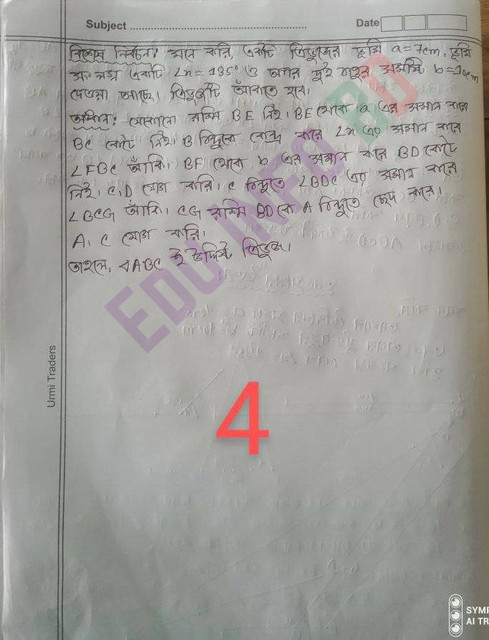# SSC 2022 9th Week Mathematics Assignment Solution

SSC 2022 ninth week maths assignment questions have been published for SSC candidates of 2022. SSC candidates of 2022 will be able to continue their educational activities by solving the 9th week math assignment. Due to the ongoing Covid-19 epidemic, the ninth week of Mathematical Assignment has been published to bring students fully involved in learning activities and continuous assessment on the basis of reorganized curriculum as directed by the Ministry of Education. The ninth week of Mathematical Assignment will start from 26th January 2022. The ninth week math assignment has been published for the SSC candidates of 2022 and the students will also hand over the answers to the teacher at their own risk as soon as possible.

## SSC 2022 9th Week Mathematics Assignment Answer

In various notifications published by the Ministry of Education, all the education systems of the country are being conducted in different ways. Following different processes at different times, the Ministry of Education has continued all educational activities across the country. Following this, SSC 2022 Maths Assignment has been published on 25th January, 2022. We have published the instructions regarding SSC 2022 Maths Assignment Answer on our website. The Ministry of Education has arranged assignments during this epidemic for the students to become proficient in mathematics. Sample answers to the assignment are published on our website. By looking at this answer students will get ideas on how to solve the assignment. You can download SSC 2022 Maths Assignment Answer from our website as a sample.

## SSC Mathematics Ninth Week Assignment Solution 2022

Psychology Second Paper has been published for the students participating in SSC Exam 2022. The ninth week math assignment has been published in the math book this time. SSC 2022 Mathematics is basically for all the candidates who have studied in all the departments in the school. Mathematics is an important subject in all departments. This time the assignment has been published about mathematics. SSC 2022 Math Assignment Solutions is available as a sample answer on our website.

Mathematical assignment work

Draw 60 °, 75 ° and 135 ° using a pencil compass
Measure and record the length and width of your rectangular reading table

## Learning Outcome of Ninth Week SSC 2022 Mathematics Assignment

• Be able to draw quadrilateral trapezium using the given data
• Be able to draw triangles using the given data

## SSC 2022 Mathematics Ninth Week Assignment Question## SSC 2022 Math Assignment Solution Guide

• Now draw a proportional figure on the surface of a trapezium shaped table whose two parallel arms will be equal to the length and width of your rectangular reading table and one of the two adjacent smaller sides of the trapezium (the width of the table) will complement 75 and the other 135. (Example 3 page number 147 of the textbook will help. If necessary, extend the smaller arm and try a parallelogram on it.)
• ABCD Draw a ratio of a quadrilateral. Where AB = length of table, BC = width of table,
• Draw a triangle whose ground is equal to the width of your table. One angle adjacent to the ground is 135 and the sum of the other two arms is equal to the length of your table. (Edit 1 of page 138 of the textbook will follow)

Students will solve SSC 2022 Maths Assignment by following their own instructions. The ninth week SSC 2022 Math Assignment Solution Sample has been published on our website. If you understand the solution of this assignment well, you will be able to answer the assignment accordingly.

## SSC 2022 Mathematics 9th Week Assignment Answer### Check Also

1.2.